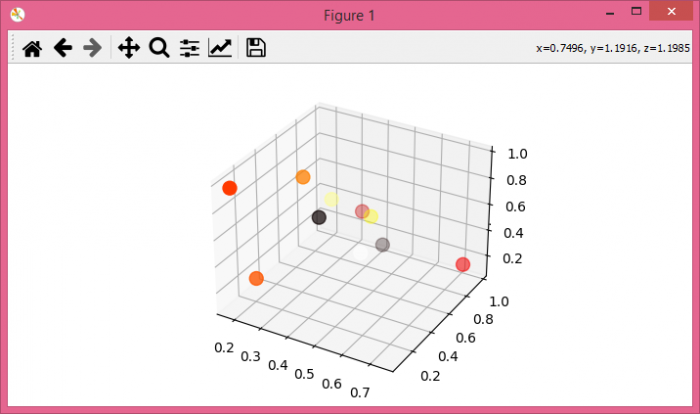# How to get the properties of a picked object in mplot3d (matplotlib + python)?

To get the properties of picked objects in matplotlib 3d, we can take the following steps.

## Steps

• Set the figure size and adjust the padding between and around the subplots.

• Create a new figure or activate an existing figure.

• Add an 'ax' to the figure as part of a subplot arrangement.

• Make a scatter plot of random data points.

• Bind the function *pick_event_method* to the event *pick_event*.

• Print x, y and z coordinates of the event.

• To display the figure, use Show() method.

## Example

import matplotlib.pyplot as plt
import numpy as np

plt.rcParams["figure.figsize"] = [7.00, 3.50]
plt.rcParams["figure.autolayout"] = True

fig = plt.figure()

# Scatter plot
ax.scatter(np.random.rand(10), np.random.rand(10), np.random.rand(10), c=np.random.rand(10),
cmap='hot', picker=5, s=100)

# pick_event_method
def pick_event_method(event):
ind = event.ind
x, y, z = event.artist._offsets3d
print(x[ind], y[ind], z[ind])

# Connect pick_event_method with pick_event
fig.canvas.mpl_connect('pick_event', pick_event_method)

plt.show()

## Output

It will produce the following output −Now, click the objects from the plot and it will display the coordinates of those points on the console.

0.29471404722373373 0.7272382336952506 0.551701540876738
0.7393059098968329 0.880733225356321 0.20733995579556608
0.4055966753557102 0.9709122739514328 0.10116103589732084
0.2781962334047674 0.48531626106129566 0.8573607199598575